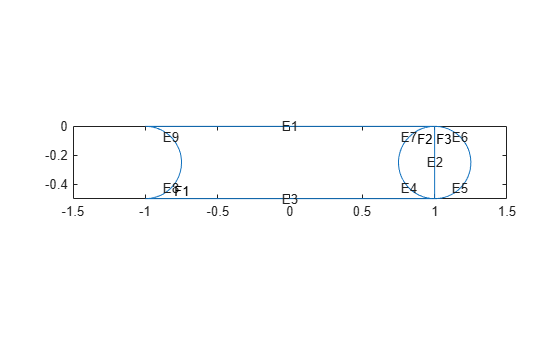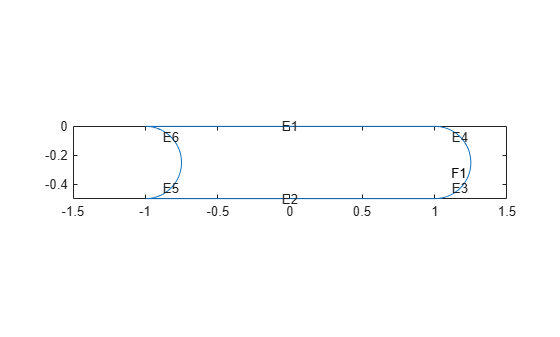Documentation

## 2-D Geometry Creation at Command Line

### Three Elements of Geometry

To describe your geometry through Constructive Solid Geometry (CSG) modeling, use three data structures.

1. A matrix whose columns describe the basic shapes. When you export geometry from the PDE Modeler app, this matrix has the default name `gd` (geometry description). See Create Basic Shapes.

2. A matrix whose columns contain names for the basic shapes. Pad the columns with zeros or 32 (blanks) so that every column has the same length. See Create Names for the Basic Shapes.

3. A set of characters describing the unions, intersections, and set differences of the basic shapes that make the geometry. See Set Formula.

### Create Basic Shapes

To create basic shapes at the command line, create a matrix whose columns each describe a basic shape. If necessary, add extra zeros to some columns so that all columns have the same length. Write each column using the following encoding.

Circle

RowValue
`1``1` (indicates a circle)
`2`x-coordinate of circle center
`3`y-coordinate of circle center
`4`Radius (strictly positive)

Polygon

RowValue
`1``2` (indicates a polygon)
`2`Number of line segments `n`
`3` through `3+n-1`x-coordinate of edge starting points
`3+n` through `2*n+2`y-coordinate of edge starting points

### Note

Your polygon cannot contain any self-intersections. To check whether your polygon satisfies this restriction, use the `csgchk` function.

Rectangle

RowValue
`1``3` (indicates a rectangle)
`2``4` (number of line segments)
`3` through `6`x-coordinate of edge starting points
`7` through `10`y-coordinate of edge starting points

The encoding of a rectangle is the same as that of a polygon, except that the first row is `3` instead of `2`.

Ellipse

RowValue
`1``4` (indicates an ellipse)
`2`x-coordinate of ellipse center
`3`y-coordinate of ellipse center
`4`First semiaxis length (strictly positive)
`5`Second semiaxis length (strictly positive)
`6`Angle in radians from x axis to first semiaxis

For example, specify a matrix that has a rectangle with a circular end cap and another circular excision. First, create a rectangle and two adjoining circles.

```rect1 = [3 4 -1 1 1 -1 0 0 -0.5 -0.5]; C1 = [1 1 -0.25 0.25]; C2 = [1 -1 -0.25 0.25];```

Append extra zeros to the circles so they have the same number of rows as the rectangle.

```C1 = [C1;zeros(length(rect1) - length(C1),1)]; C2 = [C2;zeros(length(rect1) - length(C2),1)];```

Combine the shapes into one matrix.

`gd = [rect1,C1,C2];`

### Create Names for the Basic Shapes

In order to create a formula describing the unions and intersections of basic shapes, you need a name for each basic shape. Give the names as a matrix whose columns contain the names of the corresponding columns in the basic shape matrix. Pad the columns with 0 or 32 if necessary so that each has the same length.

One easy way to create the names is by specifying a character array whose rows contain the names, and then taking the transpose. Use the `char` function to create the array. `char` pads the rows as needed so all have the same length. Continuing the example, give names for the three shapes.

```ns = char('rect1','C1','C2'); ns = ns';```

### Set Formula

Obtain the final geometry by writing a set of characters that describes the unions and intersections of basic shapes. Use `+` for union, `*` for intersection, `-` for set difference, and parentheses for grouping. `+` and `*` have the same grouping precedence. `-` has higher grouping precedence.

Continuing the example, specify the union of the rectangle and `C1`, and subtract `C2`.

`sf = '(rect1+C1)-C2';`

### Create Geometry and Remove Face Boundaries

After you have created the basic shapes, given them names, and specified a set formula, create the geometry using `decsg`. Often, you also remove some or all of the resulting face boundaries. Completing the example, combine the basic shapes using the set formula.

`[dl,bt] = decsg(gd,sf,ns);`

View the geometry with and without boundary removal.

```pdegplot(dl,'EdgeLabels','on','FaceLabels','on') xlim([-1.5,1.5]) axis equal```Remove the face boundaries.

```[dl2,bt2] = csgdel(dl,bt); % removes face boundaries figure pdegplot(dl2,'EdgeLabels','on','FaceLabels','on') xlim([-1.5,1.5]) axis equal```### Decomposed Geometry Data Structure

A decomposed geometry matrix has the following encoding. Each column of the matrix corresponds to one boundary segment. Any 0 entry means no encoding is necessary for this row. So, for example, if only line segments appear in the matrix, then the matrix has 7 rows. But if there is also a circular segment, then the matrix has 10 rows. The extra three rows of the line columns are filled with 0.

RowCircleLineEllipse
`1``1``2``4`
`2`Starting x coordinateStarting x coordinateStarting x coordinate
`3`Ending x coordinateEnding x coordinateEnding x coordinate
`4`Starting y coordinateStarting y coordinateStarting y coordinate
`5`Ending y coordinateEnding y coordinateEnding y coordinate
`6`Region label to left of segment, with direction induced by start and end points (`0` is exterior label)Region label to left of segment, with direction induced by start and end points (`0` is exterior label)Region label to left of segment, with direction induced by start and end points (`0` is exterior label)
`7`Region label to right of segment, with direction induced by start and end points (`0` is exterior label)Region label to right of segment, with direction induced by start and end points (`0` is exterior label)Region label to right of segment, with direction induced by start and end points (`0` is exterior label)
`8`x coordinate of circle center0x coordinate of ellipse center
`9`y coordinate of circle center0y coordinate of ellipse center
`10`Radius0Length of first semiaxis
`11`00Length of second semiaxis
`12`00Angle in radians between x axis and first semiaxis
##### SupportGet trial now